Geo Blossom Latex Balloon 6" – Directwells.comGEO BLOSSOM LATEX BALLOONS 6"

# Geo Blossom Latex Balloon 6"

Sort by:
##### 50 Qualatex White Geo Blossom Latex Balloons 6"

Geo Blossom

\$ 12.99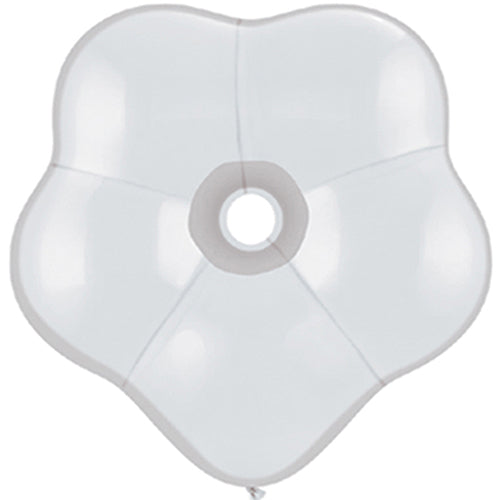### 50 Qualatex White Geo Blossom Latex Balloons 6"

\$ 12.99

50 Qualatex white Geo Blossom Latex Balloons 6". There are 50 latex balloons per bag.

##### 50 Qualatex Red Geo Blossom Latex Balloons 6"

Geo Blossom

\$ 14.99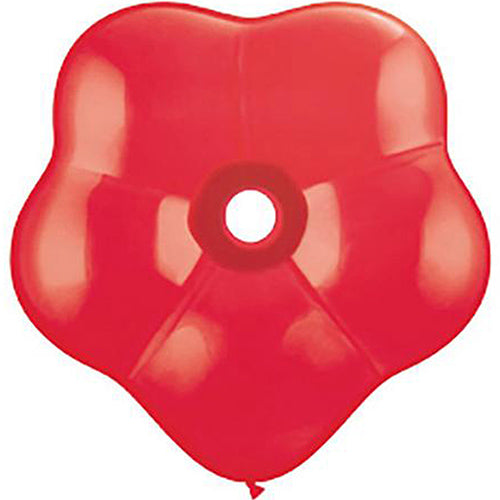### 50 Qualatex Red Geo Blossom Latex Balloons 6"

\$ 14.99

50 Qualatex red Geo Blossom Latex Balloons 6". There are 50 latex balloons per bag.

##### 50 Qualatex Pink Geo Blossom Latex Balloons 6"

Geo Blossom

\$ 14.99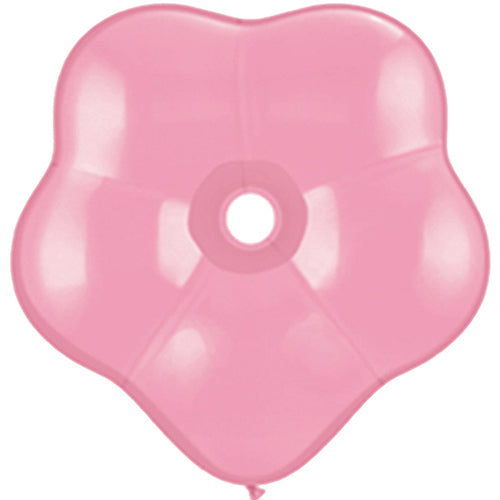### 50 Qualatex Pink Geo Blossom Latex Balloons 6"

\$ 14.99

50 Qualatex Pink Geo Blossom Latex Balloons 6". There are 50 latex balloons per bag.

##### 50 Qualatex Yellow Geo Blossom Latex Balloons 6"

Geo Blossom

\$ 12.99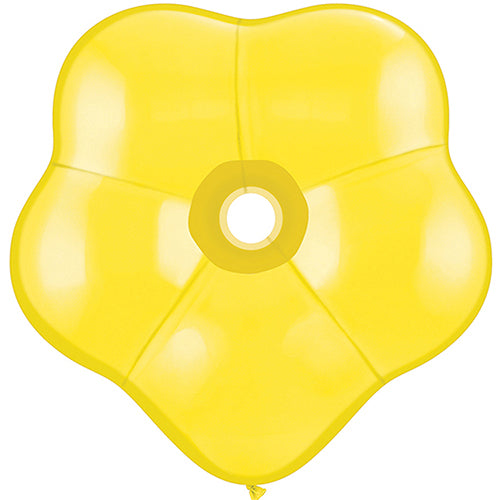### 50 Qualatex Yellow Geo Blossom Latex Balloons 6"

\$ 12.99

50 Qualatex Yellow Geo Blossom Latex Balloons 6". There are 50 latex balloons per bag.

##### 50 Qualatex Spring Lilac Geo Blossom Latex Balloons 6"

Geo Blossom

\$ 12.99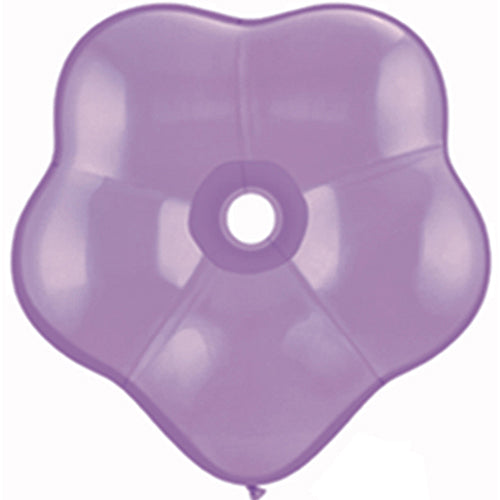### 50 Qualatex Spring Lilac Geo Blossom Latex Balloons 6"

\$ 12.99

50 Qualatex spring lilac Geo Blossom Latex Balloons 6". There are 50 latex balloons per bag.

##### 50 Qualatex Lime Green Geo Blossom Latex Balloons 6"

Geo Blossom

\$ 14.99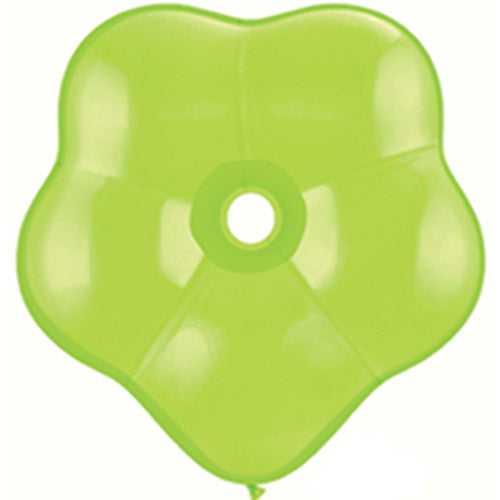### 50 Qualatex Lime Green Geo Blossom Latex Balloons 6"

\$ 14.99

50 Qualatex lime green Geo Blossom Latex Balloons 6". There are 50 latex balloons per bag.

##### 50 Qualatex Dark Blue Geo Blossom Latex Balloons 6"

Geo Blossom

\$ 14.99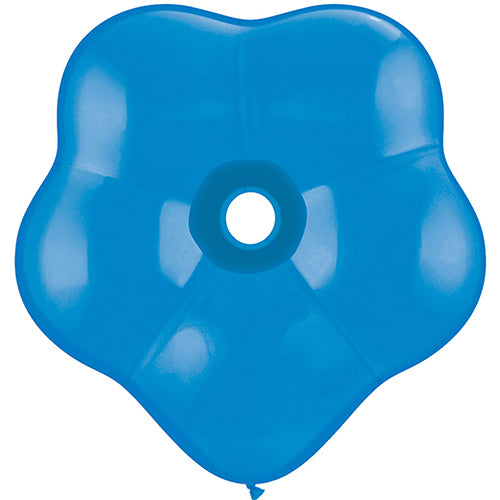### 50 Qualatex Dark Blue Geo Blossom Latex Balloons 6"

\$ 14.99

50 Qualatex dark blue Geo Blossom Latex Balloons 6". There are 50 latex balloons per bag.